Journal of Multimedia Information System
Korea Multimedia Society
Section B

# Curvature and Histogram of oriented Gradients based 3D Face Recognition using Linear Discriminant Analysis

Yeunghak Lee1,*
1Kyungwoon Univ. 55 Indoek-ri, Sandong-myeon, Gumi, Korea, +82-54-479-1215, annaturu@ikw.ac.kr.
*Corresponding Author: Yeunghak, Lee, Kyungwoon Univ. 55 Indoek-ri, Sandong-myeon, Gumi, Korea, +82-54-479-1215, annaturu@ikw.ac.kr.

© Copyright 2015 Korea Multimedia Society. This is an Open-Access article distributed under the terms of the Creative Commons Attribution Non-Commercial License (http://creativecommons.org/licenses/by-nc/4.0/) which permits unrestricted non-commercial use, distribution, and reproduction in any medium, provided the original work is properly cited.

Received: Mar 31, 2015 ; Revised: Apr 20, 2015 ; Accepted: May 10, 2015

Published Online: Mar 31, 2015

## Abstract

This article describes 3 dimensional (3D) face recognition system using histogram of oriented gradients (HOG) based on face curvature. The surface curvatures in the face contain the most important personal feature information. In this paper, 3D face images are recognized by the face components: cheek, eyes, mouth, and nose. For the proposed approach, the first step uses the face curvatures which present the facial features for 3D face images, after normalization using the singular value decomposition (SVD). Fisherface method is then applied to each component curvature face. The reason for adapting the Fisherface method maintains the surface attribute for the face curvature, even though it can generate reduced image dimension. And histogram of oriented gradients (HOG) descriptor is one of the state-of-art methods which have been shown to significantly outperform the existing feature set for several objects detection and recognition. In the last step, the linear discriminant analysis is explained for each component. The experimental results showed that the proposed approach leads to higher detection accuracy rate than other methods.

Keywords: Curvature; Histogram of oriented gradients; LDA; Face recognition

## I. INTRODUCTION

The ability to recognize a person is a task that human risibly performs but one that computers to date have been unable to perform robustly. For the automatic user identification or surveillance, various researches using human face information are performed using biometric information (fingerprint, face, iris, voice, vein, etc.) . In a biometric identification system, the face recognition with a non-touch style is a very challenging research area, next to the fingerprint. Many different human face recognition algorithms have been developed and motivated by the face recognition is non-touch style, for the last 30 years . There are several problems that are easily influenced by lighting illuminance and encountered difficulties when the face is angled away from the camera, especially in two dimensional face recognition systems. To solve these problems, a 3D face recognition system using stereo matching, laser scanner and etc. has been developed [2-3].

Broadly speaking, two approaches have emerged to recognize the face. One type employs the facial feature based approach and the other is the area based approach [4-5]. Recently, as the 3D system is being cheaper, smaller, and faster than it used to be, the research on the 3D face image is performed more actively [2-3]. Many researchers have used differential geometry tools for computing the curvatures in 3D face . Hiromi et al.  treated the problem of 3D shape recognition with rigid free-form surfaces. Each face in the input images and the model database are represented as an Extended Gaussian Image (EGI), constructed by mapping principal curvatures and their directions. Gordon  presented the study of face recognition based on depth and curvature features. To find face specific descriptors, he used the curvatures of the face. Comparison of the two faces was made based on the relationship between the spacing of the features. Lee and Milios  extracted the convex regions of the face by segmenting the range of the images based on the sign of the mean and Gaussian curvature at each point. For each of these convex regions, the Extended Gaussian Image (EGI) was extracted and then used to match the facial features of the two face images. One of the most successful techniques of the face recognition as statistical method is principal component analysis (PCA), specifically eigenfaces [10-11]. However, it is not ideal for classification purpose as it retains unwanted variations occurring due to diversified face shape and face poses. To overcome this problem, proposed method was an enhancement known as the Fisherface method, or Fisher’s linear discriminant (FLD), linear discriminant analysis (LDA) . Especially, Zhao et al.  suggested multiple feature domains based on the face recognition using the FLD through the dividing (4 types) of an original image.

The single characteristics, widely used to detect pedestrians and objects, are incorporated edge , appearance , local binary pattern (LBP) , histogram of oriented gradients (HOG) , Haar-like  and wavelet coefficient . HOG characteristics or improved HOG characteristics are widely utilized in the methods for the recognizing pedestrians by using the automobile vision. Zhu et al.  applied the HOG characteristics based on variable block size to improve detection speed. Further, Watanabe et al.  utilized co-occurrence HOG characteristics, and Wang et al.  utilized HOG-LBP human detection to improve detection accuracy.

In this paper, a novel face recognition method is introduced using the face curvatures and histogram of gradients oriented algorithm presented well personal characteristics and reduced the feature dimension. Moreover, the normalized facial pose images using SVD are considered to improve the recognition rate, as the preprocessing.

This paper is organized as follow. In section II, this paper explains the face pose normalization to improve the recognition rate. Section III describes the face surface curvature and HOG including personal feature information. To classify the person, linear discriminant analysis is introduced in section IV. The results of their evaluation and a detailed performance analysis are presented in section V. Section VI concludes this paper.

## II. Face Pose Normalization 

The nose is protruded shape and located in the middle of the face. So it can be used as the reference point, firstly we tried to find the nose tip using the iterative selection method, after extraction of the face from the 3D face image . Usually, face recognition systems suffer from drastic losses in performance when the face is not correctly oriented. The normalization process proposed here is a sequential procedure that aims at putting the face shapes in a standard spatial position. The processing sequence is panning, rotation and tilting.

2.1 Find Nose Tip

In preprocessing, a given 3D image is divided into face and background areas. Useless areas around the head and clothing parts contain too much erroneous data to process. Firstly, the nose tip is found by the iteration selection method(ISM) after using Sobel processing, as shown in Figure 1 (b).

(1)

where M and N represent the size of the image. And P stands for the depth image and B is the binary image. In the result area, the new threshold value is calculated by using an average, and the new area is extracted by using P. By the repeated processes (1), the nose tip point can be found as the highest value on the range face. The results are shown in Figure 1 (c).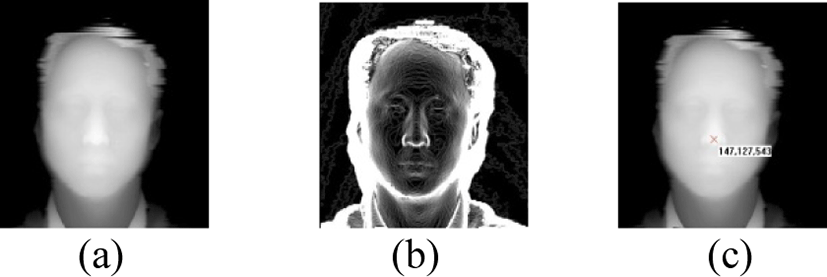Fig. 1. Preprocessing to find the nose tip using ISM (a) Original image, (b) Sobel processed image (c) The result of nose tip finding
2.2 Face Normalization

In feature recognition of 3D faces, one has to take into consideration the obtained frontal posture. Face recognition systems suffer from drastic losses in performance when the face is not correctly oriented . The normalization process proposed here is a sequential procedure that aims at putting the face shapes in a standard spatial position. Obtained face poses consist of front, right rotation, left rotation, right panning, left panning, up tilt, and down tilt.

If the pose transform matrix is AP (P = RL, RR, PL, PR, TU, TD), AP can be calculated with equation (2).

(2)

where CPF is transformed front pose for each pose, CP means left rotation (RL), right rotation (RR), left panning (PL), right panning (PR), up tilt (TU), and down tilt (TD), which has PCA coefficient matrix for all the training sets. C+ is the pseudo inverse matrix using Singular Value Decomposition (SVD) for the matrix . The reason why we have to use is that it is difficult to get the reverse matrix from the matrix which has no square matrix. The equation (3) explains the brief algorithm for the pose transform.

(3)

## III. Surface Curvature and HOG

3.1 Surface Curvature

For each data point on the facial surface, the principal, Gaussian and mean curvatures are calculated and the signs of those (positive, negative and zero) are used to determine the surface type at every point. The z(x, y) image represents a surface where the individual Z-values are surface depth information. The curvatures and related variables are computed for the pixel at location . Each pixel has an intensity value, a gray ton value or a depth value z(x, y). These intensity values define a surface in a three dimensional space as shown in Figure 2.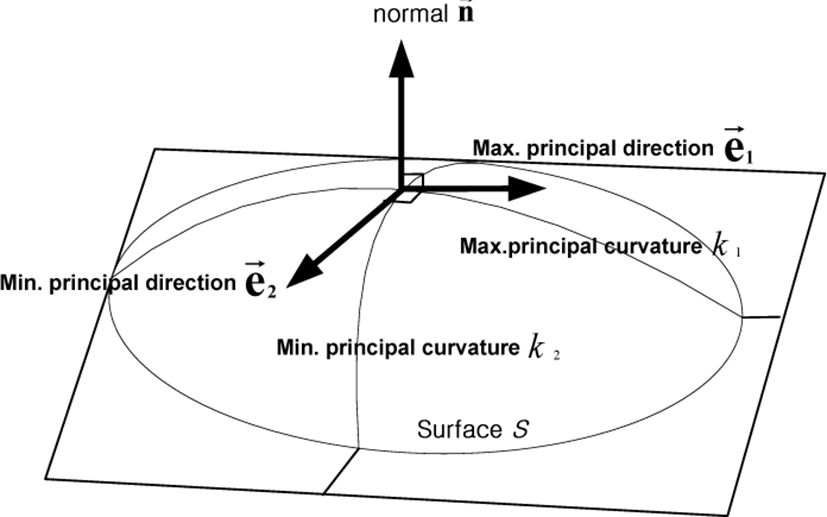Fig. 2. Principal curvatures {k1, k2} and directivity $\left\{{\stackrel{\to }{e}}_{1},{\stackrel{\to }{e}}_{2}\right\}$ at a point on the surface.

Here, x and y are the two spatial coordinates. We now closely follow the formalism introduced by Peet and Sahota , and specify any point on the surface by its position vector:

$R\left(x,y\right)=xi+yj+z\left(x,y\right)k$
(4)

The first fundamental form of the surface is the expression for the element of arc length of curves on the surface which pass through the point under consideration. It is given by:

$I=d{s}^{2}=dR\cdot dR=Ed{x}^{2}+2Fdxdy+Gd{y}^{2}$
(5)

where

$E=1+{\left(\frac{\partial z}{\partial x}\right)}^{2},\text{\hspace{0.17em}}\text{\hspace{0.17em}}F=\frac{\partial z}{\partial x}\frac{\partial z}{\partial y},G=1+{\left(\frac{\partial z}{\partial y}\right)}^{2}$
(6)

The second fundamental form arises from the curvature of these curves at the point of interest and in the given direction:

$II=ed{x}^{2}+2fdxdy+gd{y}^{2}$
(7)

where

$e=\frac{{\partial }^{2}z}{\partial {x}^{2}}\Delta ,f=\frac{{\partial }^{2}z}{\partial x\partial y}\Delta ,g=\frac{{\partial }^{2}z}{\partial {y}^{2}}\Delta$
(8)

and

$\Delta ={\left(EG-{F}^{2}\right)}^{-1/2}$
(9)

Casting the above expression into matrix form with;

$V=\left(\frac{dx}{dy}\right),\text{\hspace{0.17em}}A=\left(\begin{array}{cc}E& F\\ F& G\end{array}\right),\text{\hspace{0.17em}}B=\left(\begin{array}{cc}e& f\\ f& g\end{array}\right)$
(10)

the two fundamental forms become:

$I={V}^{t}AV\text{\hspace{0.17em}}I={V}^{t}BV$
(11)

Then the curvature of the surface in the direction defined by V is given by:

$k=\frac{{V}^{t}BV}{{V}^{t}AV}$
(12)

Extreme values of k are given by the solution to the eigenvalue problem:

$\left(B-kA\right)V=0$
(13)

or

$|\begin{array}{cc}e-kE& f-kF\\ f-kF& g-kG\end{array}|=0$
(14)

which gives the following expressions for k1 and k2, the minimum and maximum curvatures, respectively:

${k}_{1}=\left\{gE-2Ff+Ge-\left[{\left(gE+Ge-2Ff\right)}^{2}-4\left(eg-{f}^{2}\right)\left(EG-{F}^{2}\right){\right]}^{1/2}\right\}/2\left(EG-{F}^{2}\right)$
(15)
${k}_{2}=\left\{gE-2Ff+Ge+\left[{\left(gE+Ge-2Ff\right)}^{2}-4\left(eg-{f}^{2}\right)\left(EG-{F}^{2}\right){\right]}^{1/2}\right\}/2\left(EG-{F}^{2}\right)$
(16)

Here we have ignored the directional information related to k1 and k2, and chosen k2 to be the larger of the two. For the present work, however, this has not been done. The two quantities, k1 and k2, are invariant under rigid motions of the surface. This is a desirable property for us since the cell nuclei have no predefined orientation on the slide (the x – y plane).

The Gaussian curvature K and the mean curvature M are defined by

$K={k}_{1}{k}_{2},\text{\hspace{0.17em}}M=\left({k}_{1}{k}_{2}\right)/2$
(17)

which gives k1 and k2, the minimum and maximum curvatures, respectively. It turns out that the principal curvatures, k1 and k2, and Gaussian are best suited to the detailed characterization for the facial surface, as illustrated in Fig. 1. For the simple facet model of the second order polynomial of the form, i.e. a 3 by 3 window implementation in our range images, the local region around the surface is approximated by a quadric

$z\left(x,y\right)={a}_{00}+{a}_{10}x+{a}_{01}y+{a}_{01}y+{a}_{20}{x}^{2}+{a}_{02}{y}^{2}+{a}_{11}xy$
(18)

and the practical calculation of principal and Gaussian curvatures is extremely simple.

HOG  converts the distribution directions of brightness for a local region into a histogram to express them in feature vectors, which is utilized to express the shape characteristics of an object. And it is influenced a little from an effect of illumination by converting the distribution of near pixels for a local region into a histogram, and has a strong feature for a geometric change of local regions. The following is a detailed explanation on how HOG description is calculated.

Value of gradient at every image pixel is calculated by derivatives fx and fy in x and y direction by convolving the filter mask [-1 0 1] and [-1 0 1]T. Refer equation (19) and (20).

${f}_{x}=I\otimes \left[\begin{array}{ccc}-1& 0& 1\right]\end{array}$
(19)
${f}_{y}=I\otimes \left[{\begin{array}{ccc}-1& 0& 1\right]\end{array}}^{T}$
(20)

where I is an example gray scale image and ⊗ is the convolution operation. The gradient magnitudes m(x, y) and orientation direction θ(x, y) for each pixel are calculated by

$m\left(x,y\right)=\sqrt{{f}_{x}{\left(x,y\right)}^{2}+{f}_{y}{\left(x,y\right)}^{2}}$
(21)
$\theta \left(x,y\right)=\mathrm{arctan}\left(\frac{{f}_{y}\left(x,y\right)}{{f}_{x}\left(x,y\right)}\right)$
(22)
3.2.2 Orientation Binning

This stage defines production of an encoding that is sensitive to local image content. The image windows are divided into 8x8 rectangular small spatial regions call cells, as shown in figure 1 (c). Similar to , we used unsigned gradients in conjunction with nine bins for every cell (a bin corresponds to 20°). The 8×8 cell magnitude pixels are accumulated in one of the nine bins according to their orientation direction. Figure 1 (c) depicts a graphical representation on how the gradient angle range is binned in its respective cell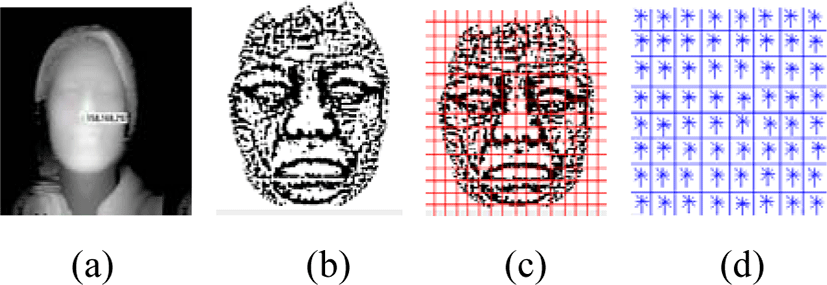Fig. 3. The example of 3D faces HOG normalization. (a) 3D facial image (b) Mean curvature (c) cell image (10x10 pixels) (d) normalization by blocks (a block is 2×2 cells).
3.2.3 Block Normalization

Directional histograms for brightness prepared in each of the cells were normalized as a block of 3×3 cells. This is performed by grouping cells in larger spatial regions called blocks. Characteristic quantities (9 dimensions) of row i, column j, Cell (i, j) are expressed as Fi,j=[F1, F1, … ,F9]. The characteristic quantities of k’th block (81 dimensions) may be expressed as:

${B}_{k}=\left[{F}_{ij},{F}_{i+1j},{F}_{i+2j},{F}_{ij+1},{F}_{ij+2}{F}_{i+1j+1},{F}_{i+2j+1},{F}_{i+1j+2},{F}_{i+2j+2}\right]$
(23)

Normalization processes are summarized in figure 1 (d), where a movement of block is based on the fact that it is moved to the right side and to the lower side by one each cell. And the feature vectors are saved by concatenation method. The overlapping process is done to ensure the important features of each cell. And the normalized characteristic vectors are given by

$\Pi =\frac{{B}_{k}}{\sqrt{{‖{B}_{k}‖}^{2}+{\epsilon }^{2}}}\left(\epsilon =1\right)$
(24)

For example, the dimension numbers for the height and width of an input image are 128x64 pixels, the dimension number of the histogram is 9, the size of cell is 8, and the size of block is 3, then the calculated number of HOG feature vectors with 6804 dimension is obtained.

## IV. Linear Discriminant Analysis

LDA searches for the projection axes on which the face images of different are far from each other (similar to PCA), and at the same time where the images of the same class are close from each other. It is class specific method in the scene that it can represent data in form which is more useful for classification. Given a set of N images {x1,x2,…,xN}, assuming each image belongs to one of the c classes {X1,X2,…,Xc}, and LDA selects a linear transformation matrix W in such a way that the ratio of the between-class scatter and the within-class scatter is maximized. Mathematically, the between-class scatter matrix and the within-class scatter matrix are defined as

$\begin{array}{ll}{S}_{B}\hfill & =\sum _{i=1}^{c}{N}_{i}\left({\mu }_{i}-\mu \right){\left({\mu }_{i}-\mu \right)}^{T}\hfill \\ {S}_{W}\hfill & =\sum _{i=1}^{c}\sum _{{x}_{k}\in {X}_{i}}^{}\left({x}_{k}-{\mu }_{i}\right){\left({x}_{k}-{\mu }_{i}\right)}^{T}\hfill \end{array}$
(25)

respectively, where μi denotes the mean image of class Xi and Ni denotes the number of images in the class Xi. If SW is nonsingular, LDA will find an orthonormal matrix Wopt maximizing the ratio of the determinant of the between-class scatter matrix to the determinant of the within-class scatter matrix. That is, the LDA projection matrix is represented by

${W}_{opt}=\mathrm{arg}\underset{W}{\mathrm{max}}\frac{|{W}^{T}{S}_{B}W|}{|{W}^{T}{S}_{W}W|}=\left[{w}_{1}\begin{array}{cc}& {w}_{2}\end{array}\begin{array}{cc}& \cdots \begin{array}{cc}& {w}_{m}\right]\end{array}\end{array}$
(26)

The set of the solution {wi|i=1,2,…m} is that of the generalized eigenvectors of SB and SW corresponding to the m largest eigenvalues {λi|i=1,2,…m}, i.e.,

${S}_{B}{w}_{i}={\lambda }_{i}{S}_{W}{w}_{i},i=1,2,\dots ,m$
(27)

In order to overcome the singularity of SW, PCA reduces the vector dimension before applying LDA. Each LDA feature vector is represented by the vector projections

${y}_{k}={w}_{opt}^{T}{x}_{k},k=1,2,\dots ,N$
(28)

To make classification of new face image x′, we calculate the Euclidean distance between a given image x′ and training set x that is

$d\left(x,{x}^{\prime }\right)=‖y-{y}^{\prime }‖$
(29)

## V. Experimental Results

In this study, we used a 3D laser scanner made by a 4D culture to obtain a 3D face image . A database composed of 592 images is used to compare the different strategies: used training and test set consist of 296 images, 7 poses (training: first front, right rotation, right panning, and up tilt, and test: second front left rotation, left panning, and down tilt) per person. With these 3D face images, the nose tip point is found by using contour line threshold values (for which the fiducial point is the nose tip). Images around the nose area are then extracted. To perform recognition experiments for the extracted face area, we firstly need to create two sets of images, as shown in Figure 4. After finding the nose tip, we processed the face pose normalization using the SVD. And then, the face surface curvature which is well presented for personal features was obtained. For the minimum and maximum curvature distributions are shown in Figure 5. To make face components, four face area were divided according to the areas, as shown in Figure 6: eye area (E), cheek area (C), nose area (N), and mouth area (M). From each component, LDA and Norm2 recognition results are showed in Table 1.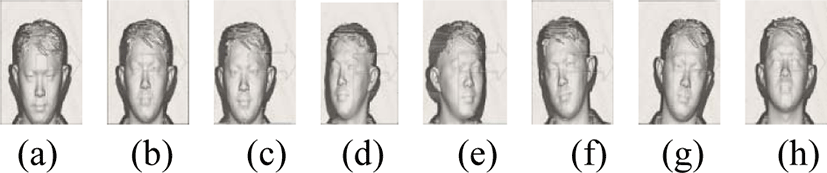Fig. 4. The example of face images; (a) The first front, (b) The second front, (c) Right Rotation, (d) Left rotation, (e) Right Panning, (f) Left Panning, (g) Up tilt, (h) Down tilt.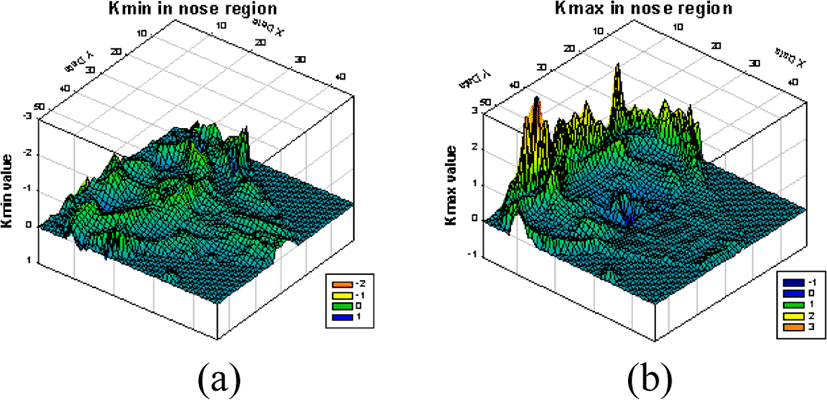Fig. 5. Distribution of minimum curvature and maximum curvature for around the nose region; (a) 3 dimensional graph for minimum curvature, (b) 3 dimensional graph for maximum curvature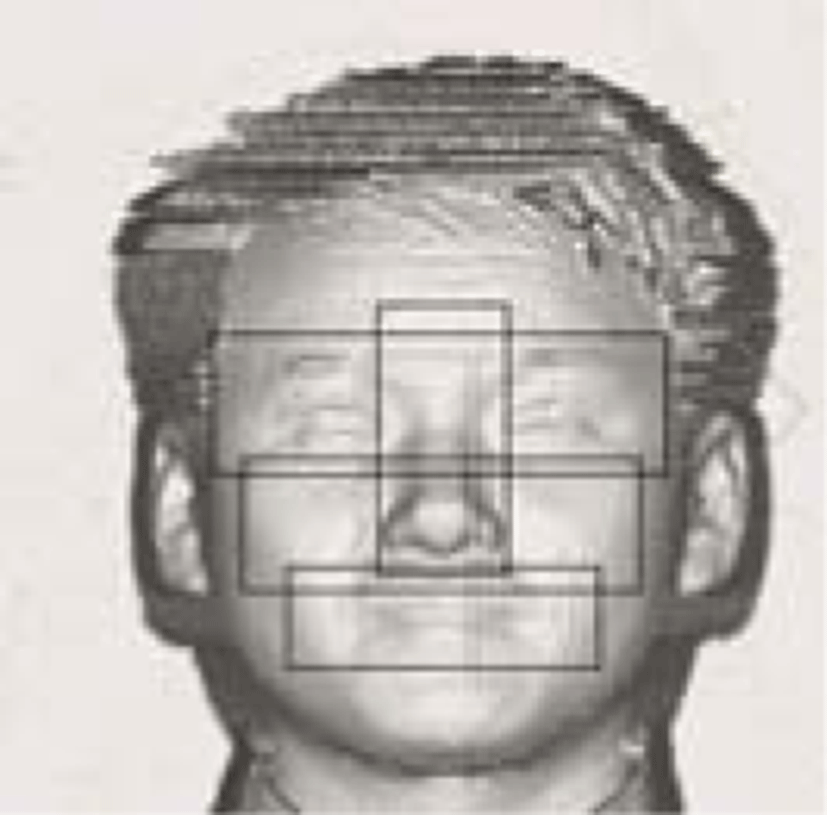Fig. 6. Displayed each component area.
Table 1. The comparision of the recognition rate (%)
Method C E M N Avg.
k1 Norm2 92.2 90.5 83.9 83.9 88.5
LDA 90.8 89.9 90.2 88.7 89.9
k2 Norm2 89.2 89.9 83.5 84.1 86.7
LDA 90.8 90.8 90.2 90.5 90.6

Generate the membership grade based on the LDA distance (di) information between the test image and the training set produced in the previous section. Using this distance, we follow the method introduced in .

${n}_{ij}=\frac{1}{1+\left({d}_{ij}/{d}_{i}\right)},\text{\hspace{0.17em}}m\left({y}_{ik}\right)=\sum _{{n}_{ij}\in {C}_{k}}\left({n}_{ij}\right)/{N}_{k}$
(30)

where i=1,2,3,4, j=1,2,…, 296, i is the number of classifier and j is the index of the training set. And Nk is the number of samples in kth class Ck.

The data sets have been extracted with the aid of LDA. The cheek part among the face components represented the highest recognition rate, 92.2% for norm2 and LDA. It shows that cheek area has outstanding curvature features, because of including the nose tip area and both sides of cheek. And also it means that it has very different shapes or surface feature for each person. In average analysis, the recognition of adapted curvature and HOG method showed 90.6% - k2, increased more than norm2 methods. Additionally, in the curvature feature, k2 showed higher recognition rate than k1.

## VI. CONCLUSION

We have introduced, in this paper, a new practical implementation of a person verification system using curvature-HOG based on the component face images. The underlying motivations of our approach originate from the observation that the surface curvature of the face has different shape based on the face components. And Ordinary HOG has two kinds of spatial region: small (cells) and large (blocks). And it is based on overlapping and dens encoding of image regions. To classify the faces, LDA and norm2 were used. It has been experimentally demonstrated that the aggregation of classifiers operating on four component face image sets generated by area based led to better classification results than norm2 method. Furthermore, we also confirmed that the curvature k2 has higher recognition rate than the k1.

From the experimental results, we proved that the process of the face recognition may use lower dimension, less parameters, and less calculation than earlier suggestion. We consider that there are many future experiments that could be done to extend this study.

## REFERENCES

.

L. C. Jain, U. Halici, I. Hayashi, and S. B. Lee, “Intelligent biometric techniques in fingerprint and face recognition,” CRC Press, 1999..

R. Chellapa, C. L. Wilson, and S. Sirohey, “Human and Machine Recognition of Faces: A Survey,” Proceeding of the IEEE, vol. 83, no. 5, pp.705-741, May, 1995.

P. W. Hallinan, G. G. Gordon, A. L. Yuille, P. Giblin, and D. Mumford, “Two and three dimensional pattern of the face,” A K Peters Ltd., 1999..

C. S. Chua, F. Han, and Y. K. Ho, “3D Human Face Recognition Using Point Signature,” Proc. of the 4th ICAFGR, pp.233-238, March, 2000..

H. T. Tanaka, M. Ikeda, and H. Chiaki, “Curvature-based face surface recognition using spherial correlation,” Proc. of the 3rd IEEE Int. Conf. on Automatic Face and Gesture Recognition, pp. 372-377, April, 1998.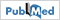.

G. G. Gordon, “Face Recognition based on depth and curvature feature,” Proc. of the IEEE Computer Society Conf. on Computer Vision and Pattern Recognition, pp. 808-810, June, 1992..

J. C. Lee and E. Milios, “Matching range image of human faces,” Proc. of the 3rd Int. Conf. on Computer Vision, pp. 722-726, December, 1990,..

M. Turk and A. Pentland, “Eigenfaces for Recognition,” Journal of Cognitive Neuroscience, vol. 3, no. 1, pp. 71-86, winter, 1991..

C. Hesher, A. Srivastava, and G. Erlebacher, “Principal Component Analysis of Range Images for Facial Recognition,” Proc. of CISST, 2002..

W. Zhang, S. Shan, W. Gao, Y. Chang, and B. Cao, “Component-based Cascade Linear Discriminant Analysis for Face Recognition,” Lecture Notes in Computer Science, vol. 3338, pp. 288-295, 2005..

D. M. Gavrila and S. Munder, “Multi-cue pedestrian detection and tracking from a moving vehicle,” International Journal of Computer Vision, vol. 73, no. 1, pp.41-59, June, 2007..

P. Sabzmeydani and G. Mori, “Detecting Pedestrians by learning shapelet features,” Proceeding of Computer Vision and Pattern Recognition, pp. 1-8, June, 2007..

T. Ahonen, A. Hadid and M. Pietikainen, “Face description with local binary patters: Application to face recognition,” IEEE Transaction Pattern Analysis and machine Intelligent. vol. 28, no. 12, pp.2037-2041, October, 2006..

N. Dalal and B. Triggs, “Histogram of Oriented Gradients for Human Detection,” IEEE Computer Vision Pattern Recognition, pp.886-893, June, 2005..

S. Pavani, D. Delgado and A. F. Frangi, “Haar-like features with optimally weighted rectangles for rapid object detection,” Pattern Recognition, vol. 43, no. 1, January, 2010..

C. Papageorgiou and T. Poggio, “A trainable system for object detection,” International Journal of Computer Vision, vol. 38, no. 1, June, 2000..

Q. Zhu, M. C. Yeh, K. T. Cheng and S. Avidan, “Fast human detection using a cascade of histograms of oriented gradients,” IEEE Conference on Computer Vision and Pattern Recognition, pp. 17-22, June 2006..

T. Watanabe, S. Ito and K. Yokoi, “Co-occurrence Histogram of Oriented Gradients for Detection Advances in Image and Video Technology,” Lecture Notes in Computer Science, vol. 5414, pp. 37-47, 2009..

X. Y. Wang, T. X. Han and S. Yan, An “HOG-LBP human detector with partial occlusion handling,” International Conference on Computer Vision, pp. 32-39, Sept. – Oct., 2009..

Y. H. Lee and D. Marshall, “Curvature based 3D component facial image recognition using fuzzy integral,” Applied Mathematics and Computation, vol. 205, pp. 815-823, 2008..

Y. H. Lee, T. S. Kim, S. H. Lee, and J. C. Shim, “New approach to two wheelers detection using Cell Comparison,” Journal of Multimedia and Information System, vol. 1, no. 1, pp. 45-53, December, 2014..

Peet, F. G., Sahota, T. S.: “Surface Curvature as a Measure of Image Texture,” IEEE Trans. PAMI, vol. 7, no. 6, pp. 734-738, January, 2009..

G. Banon, “Distinction between several subsets of fuzzy measures,” Fuzzy Sets and Systems, vol. 5, no. 4, pp. 291-305, May, 1981..

K. C. Kwak and W. Pedrycz, “Face Recognition using fuzzy integral and wavelet decomposition methos,” IEEE Transaction on System, Man, and Cybernetics, vol.34, no. 4, pp.1666-1675, April, 2004.